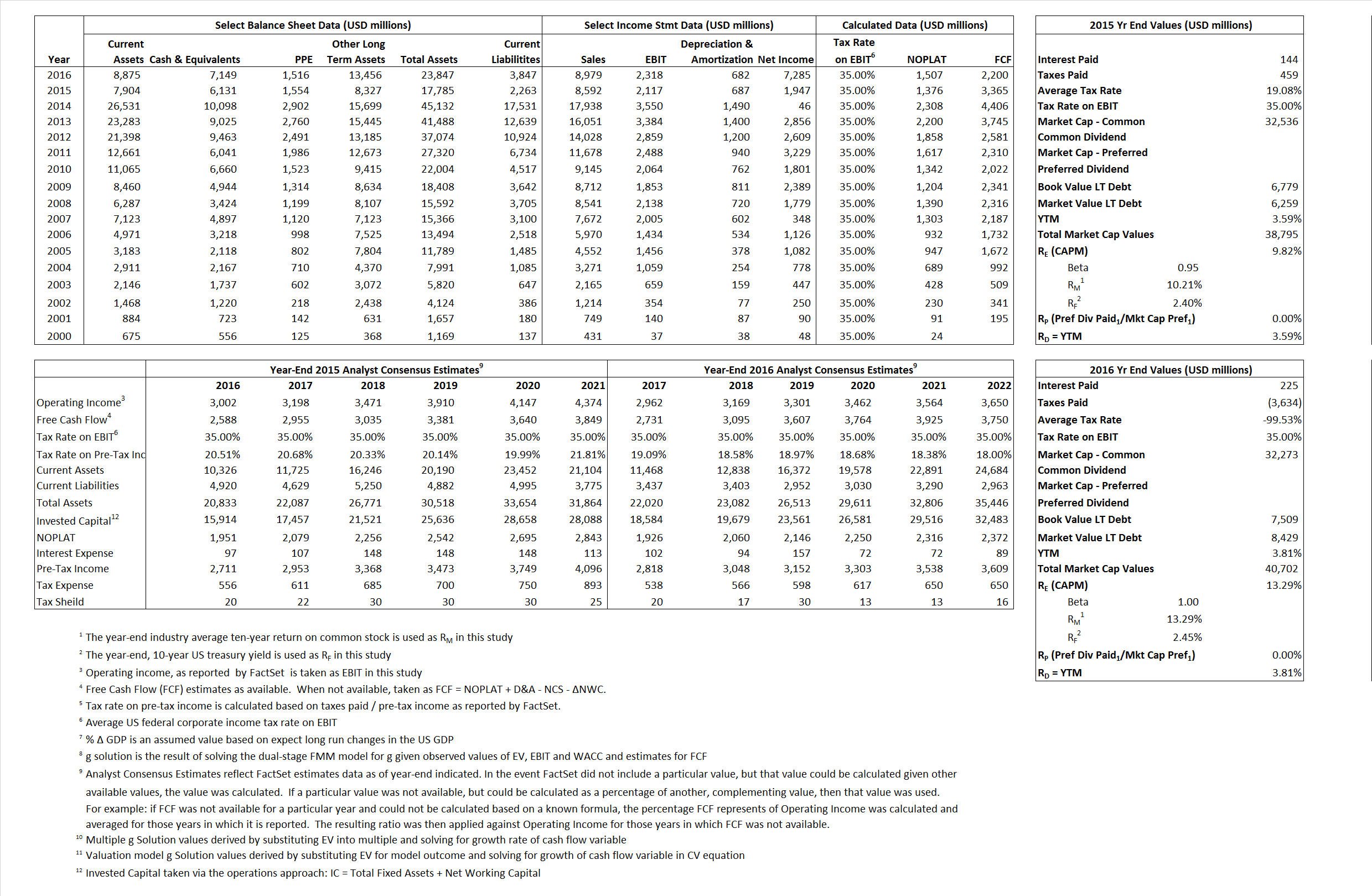# eBay Inc.

## Analyst Listing

The following analysts provide coverage for the subject firm as of May 2016:

 Broker Analyst Analyst Email Raymond James Aaron Kessler aaron.kessler@raymondjames.com Jefferies Brian Pitz bpitz@jefferies.com Bernstein Research Carlos Kirjner carlos.kirjner@bernstein.com Monness Crespi Hardt Cengiz M. Cakmak jcakmak@mchny.com The Benchmark Company, LLC Daniel L. Kurnos dkurnos@benchmarkcompany.com BMO Capital Markets Daniel Salmon dan.salmon@bmo.com Piper Jaffray Gene Munster gene.a.munster@pjc.com Wedbush Securities Gil Luria gil.luria@wedbush.com Atlantic Equities James Cordwell j.cordwell@atlantic-equities.com Cowen & Company John Blackledge john.blackledge@cowen.com Daiwa Securities Co. Ltd. Kazuya Nishimura kazuya.nishimura@us.daiwacm.com Evercore ISI Ken Sena ken.sena@evercoreisi.com Needham Kerry Rice krice@needhamco.com RBC Capital Markets Mark S. Mahaney mark.mahaney@rbccm.com Canaccord Genuity Michael Graham mgraham@canaccordgenuity.com Mizuho Securities USA Neil A. Doshi neil.doshi@us.mizuho-sc.com SunTrust Robinson Humphrey Robert S. Peck robert.peck@suntrust.com JMP Securities Ronald V. Josey rjosey@jmpsecurities.com Stifel Nicolaus Scott W. Devitt swdevitt@stifel.com FBN Securities Shebly Seyrafi sseyrafi@fbnsecurities.com Susquehanna Financial Group Shyam Patil shyam.patil@sig.com Credit Suisse Stephen Ju stephen.ju@credit-suisse.com Axiom Capital Victor Anthony vanthony@axiomcapital.com Cantor Fitzgerald Youssef H. Squali ysquali@cantor.com

## Primary Input Data## Derived Input Data

### Equational Form

Net Operating Profit Less Adjusted Taxes NOPLAT  1,376 1,507$NOPLAT\, =\, EBIT\, x\, (1 \,-\, Avg \,\,Tax\,\, Rate\,\, on\,\, EBIT)$
Free Cash Flow FCF 3,365 2,200$FCF\,=NOPLAT\,+\,Non-Cash\,Expenses-\Delta NWC\,-\,NCS$
Tax Shield TS 27 (224)$TS\,=\,Interest\,\,Paid\,\,x\,\, Avg \,\,Tax\,\,Rate\,\, on\,\, Pre-Tax\,\, Income$
Invested Capital IC 15,522 20,000$IC\,=\,Fixed\,\,Operating\,\,Assets\,\,+\,\,Net\,\, Working\,\, Capital$
Return on Invested Capital ROIC 8.87% 7.53%$ROIC\,=\,\frac { NOPLAT }{ IC }$
Net Investment NetInv (11,392) 5,160$NetInv\,=\,{ {IC}_{1}}-{{IC}_{0}}+Depreciation$
Investment Rate IR  -827.88%  342.47%$IR\,=\,\frac {NetInv}{NOPLAT}$
Weighted Average Cost of Capital
WACCMarket 8.70% 12.11%$WACC\,=\,\frac { E }{ V } { R }_{ E }\,+\,\frac { P }{ V } { R }_{ P }\,+\,\frac { D }{ V } { R }_{ D }\left( 1- Avg\,\, Tax\,\,Rate\,\,on\,\,Pre-Tax\,\,Income \right)$
WACCBook     7.91%     7.67%
Enterprise value
EVMarket 32,664  33,553$EV\,=\,Market\,\,Cap\,\,Equity\,+\,\,Long\,\,Term\,\,Debt\,-\,Cash$
EVBook     33,184    32,633
Long-Run Growth
g = IR x ROIC
-73.39%    25.80% Long-run growth rates of the income variable are used in the Continuing Value portion of the valuation models.
g = %$\Delta$ GDP     2.50%     2.50%
Margin from Operations M   24.64%   25.82%$M\,\,=\,\,\frac{EBIT}{SALES}$
Depreciation/Amortization Rate D   24.50%   22.73%$D\,\,=\,\,\frac{D+A}{EBITDA}$

## Valuation Multiple Outcomes

The outcomes presented in this study are the result of original input data, derived data, and synthesized inputs.

### model g solution

12/31/2015 12/31/2016 12/31/2015 12/31/2016 12/31/2015 12/31/2016

EV/SALES$\frac {EV}{Sales} \,= \,\frac{ROIC\, -\, g}{ROIC\,(WACC\,-\,g)}\,(1\,-\,T)\,(M)$

3.80  3.74  8.56%  18.87%  8.55%  14.43%

EV/EBITDA$\frac {EV}{EBITDA} \,= \,\frac{ROIC\, -\, g}{ROIC\,(WACC\,-\,g)}\,(1\,-\,T)\,(1\,-\,D)$

11.65 11.18 8.56% 18.87% 8.55% 14.43%

EV/NOPLAT$\frac {EV}{NOPLAT} \,= \,\frac{ROIC\, -\, g}{ROIC\,(WACC\,-\,g)}$

23.74 22.27 8.56% 18.87% 8.55% 14.43%

EV/FCFOPS$\frac {EV}{FCF_{OPS}} \,= \,\frac{ROIC\, -\, g}{ROIC\,(WACC\,-\,g)}\,(1\,-\,T)$

9.71 15.25 8.56% 18.87% 8.55% 14.43%

EV/EBIT$\frac {EV}{EBIT} \,= \,\frac{ROIC\, -\, g}{ROIC\,(WACC\,-\,g)}\,(1\,-\,T)$

15.43 14.47 8.56% 18.87% 8.55% 14.43%

EV/IC$\frac {EV}{IC} \,= \,\frac{ROIC\, -\, g}{WACC\,-\,g}$

2.10 1.68 8.56% 18.87% 8.55% 14.43%